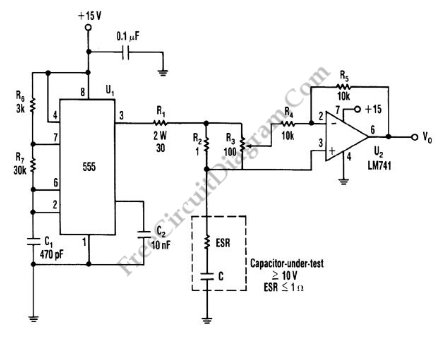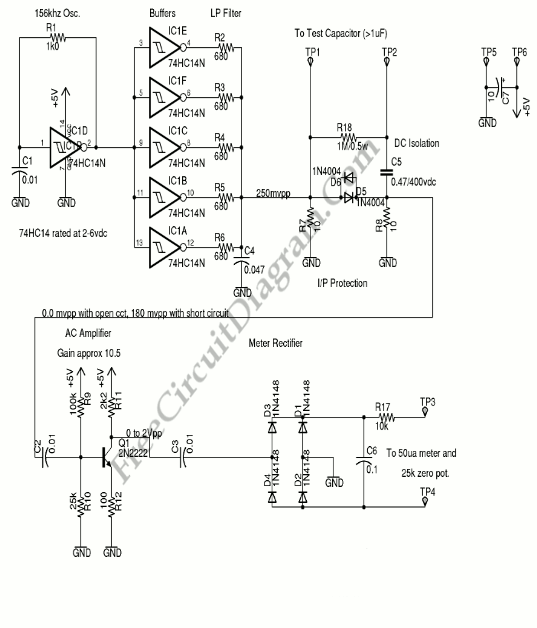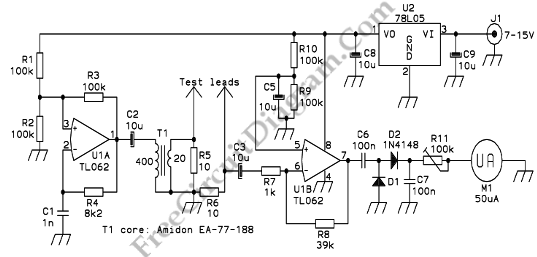## Capacitor ESR MeterThis is schematic diagram of capacitor ESR meter circuit. ESR is equivalent series resistance, and this parameter is used to express the resistance value of any electronic component that basically is not a resistor. ESR is present on many non-resistor component, such as capacitor, inductor, or semiconductor. The circuit shown in this schematic diagram is used to measure equivalent series […]

## ESR (Equivalent Series Resistance Meter) Using 74HC14ESR is Equivalent Series Resistance. ESR is one of the characteristics that defines the performance of an electrolytic capacitor. It’s highly desirable to use Low ESR in capacitor as any ripple current through the capacitor causes the capacitor to heat up due to the resistive loses. By drying out the electrolyte at an ever increasing rate, the heating accelerates the […]